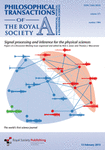# Gaussian process

Topic | v1 | created by janarez |
Description

In probability theory and statistics, a Gaussian process is a stochastic process (a collection of random variables indexed by time or space), such that every finite collection of those random variables has a multivariate normal distribution, i.e. every finite linear combination of them is normally distributed. The distribution of a Gaussian process is the joint distribution of all those (infinitely many) random variables, and as such, it is a distribution over functions with a continuous domain, e.g. time or space. A machine-learning algorithm that involves a Gaussian process uses lazy learning and a measure of the similarity between points (the kernel function) to predict the value for an unseen point from training data. The prediction is not just an estimate for that point, but also has uncertainty information—it is a one-dimensional Gaussian distribution.

##### Resources

9.0 rating 3.0 level 7.0 clarity 3.0 background – 1 rating

In this paper, we offer a gentle introduction to Gaussian processes for time-series data analysis. Th...